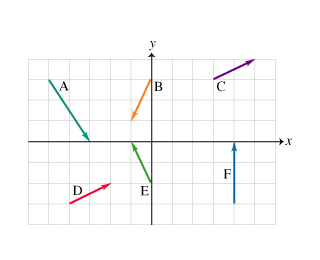# Problem: Six vectors (A to F) have the magnitudes and directions indicated in the figure.a. Which two vectors, when added, will have the largest x component?b. Which two vectors, when added, will have the largest y component?c. Which two vectors, when subtracted (i.e., when one vector is subtracted from the other), will have the largest magnitude?

###### FREE Expert Solution

Listing the components of individual vectors:

A= 2, Ay = - 3

Bx = -1, By = - 2

Cx = 2, Cy = 1

Dx = 2, Dy = 1

Ex = -1, Ey = 2

Fx = 0, Fy = 3

85% (159 ratings)###### Problem Details

Six vectors (A to F) have the magnitudes and directions indicated in the figure.a. Which two vectors, when added, will have the largest x component?

b. Which two vectors, when added, will have the largest y component?

c. Which two vectors, when subtracted (i.e., when one vector is subtracted from the other), will have the largest magnitude?